# GSEB Solutions Class 12 Statistics Part 2 Chapter 5 Differentiation Ex 5.1

Gujarat Board Statistics Class 12 GSEB Solutions Part 2 Chapter 5 Differentiation Ex 5.1 Textbook Exercise Questions and Answers.

## Gujarat Board Textbook Solutions Class 12 Statistics Part 2 Chapter 5 Differentiation Ex 5.1

Obtain the derivatives of the following functions with the help of definition:

Question 1.
f(x) = 2x + 3
Solution:
Here, f(x) = 2x + 3
∴ f(x + h) = 2 (x + h) + 3 = 2x + 2h + 3Hence, f(x) = 2x + 3 then f’(x) = 2.f(x) = x2
Solution:
Here, f(x) = x2
∴ f(x + h) = (x + h)2 = x2 + 2xh + h2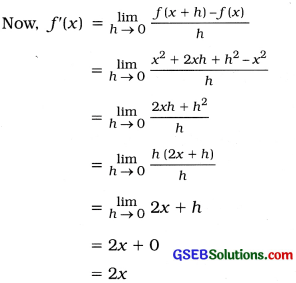Hence, f(x) = x2 then f'(x) = 2x.Question 3.
f(x) = x7
Solution:
Here, f(x) = x7
∴ f(x + h) = (x + h)7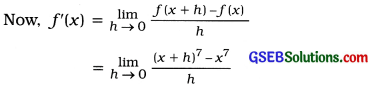Take, x + h = t, when h → 0, t → x and h = t – xHence, f(x) = x7 then f'(x) = 7x6Question 4.
f(x) = $$\frac{1}{x+1}$$, x ≠ -1
Solution:
Here, f(x) = $$\frac{1}{x+1}$$
∴ f(x + h) = f(x)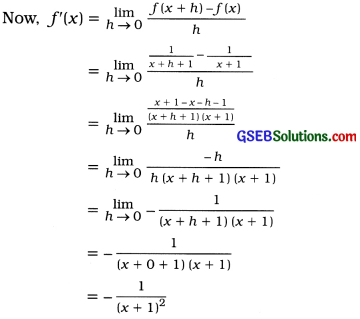Hence, f(x) = $$\frac{1}{x+1}$$ then f'(x) = $$-\frac{1}{(x+1)^{2}}$$.Question 5.
f(x) = $$\sqrt{x}$$
solution:
Here, f(x) = $$\sqrt{x}=x^{\frac{1}{3}}$$

∴ f(x + h) = $$(x+h)^{\frac{1}{3}}$$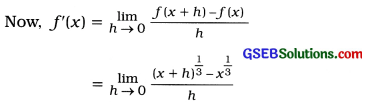Take, x + h = t, when h → 0, t → x and h = t – x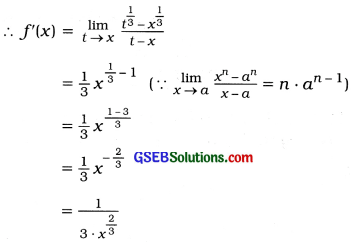Hence, f(x) = $$\sqrt{x}$$ then f'(x) = $$\frac{1}{3 \cdot x^{\frac{2}{3}}}$$.Question 6.
f(x) = 24, x ≠ $$\frac{4}{3}$$
Solution:
Here, f(x) = $$\frac{2}{3 x-4}$$

∴ f(x + h) = $$\frac{2}{3(x+h)-4}$$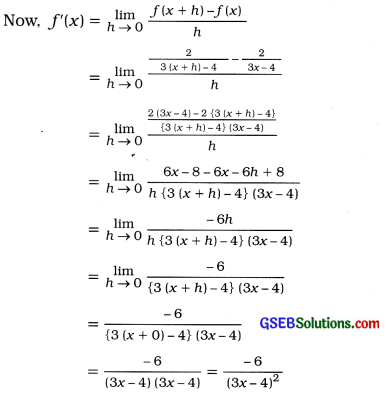Hence, f(x) = $$\frac{2}{3(x+h)-4}$$ then f’(x) = $$\frac{-6}{(3 x-4)^{2}}$$.Question 7.
f(x) = 10
Solution:
Here, f(x) = 10
∴ f(x + h) = 10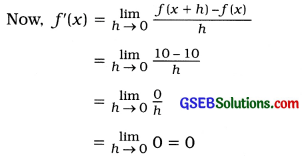Hence, f(x) = 10 then f’(x) = 0